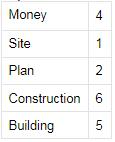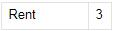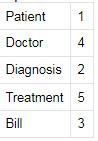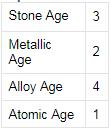Courses

# Test: Logical Sequence- 4

## 30 Questions MCQ Test UPSC CSAT Preparation | Test: Logical Sequence- 4

Description
This mock test of Test: Logical Sequence- 4 for UPSC helps you for every UPSC entrance exam. This contains 30 Multiple Choice Questions for UPSC Test: Logical Sequence- 4 (mcq) to study with solutions a complete question bank. The solved questions answers in this Test: Logical Sequence- 4 quiz give you a good mix of easy questions and tough questions. UPSC students definitely take this Test: Logical Sequence- 4 exercise for a better result in the exam. You can find other Test: Logical Sequence- 4 extra questions, long questions & short questions for UPSC on EduRev as well by searching above.
QUESTION: 1

Solution:
QUESTION: 2

Solution:
QUESTION: 3

### Directions to Solve In each of the following questions, arrange the given words in a meaningful sequence and thus find the correct answer from alternatives. Question -   Arrange the words given below in a meaningful sequence.    1. Sea    2. Rivulet    3. Ocean    4. River    5. Glacier

Solution:
QUESTION: 4

Directions to Solve

In each of the following questions, arrange the given words in a meaningful sequence and thus find the correct answer from alternatives.

Question -

Arrange the words given below in a meaningful sequence.

1. Milky way    2. Sun    3. Moon    4. Earth    5. Stars

Solution:

The words given are different planetary bodies.
They must be arranged in the increasing or decreasing order of their sizes.
So for given words, the order will be:
Moon, Earth, Sun, Stars, MilkyWay

QUESTION: 5

Directions to Solve

In each of the following questions, arrange the given words in a meaningful sequence and thus find the correct answer from alternatives.

Question -

Arrange the words given below in a meaningful sequence.

1. Doctor    2. Fever    3. Prescribe    4. Diagnose    5. Medicine

Solution:
QUESTION: 6

Directions to Solve

In each of the following questions, arrange the given words in a meaningful sequence and thus find the correct answer from alternatives.

Question -

Arrange the words given below in a meaningful sequence.

1. Reading    2. Composing    3. Writing    4. Printing

Solution:

The words given are the various stages in making of a book.
So first step is the Writing.
Next will be Composing it suitably.
so order is: 3,2,4,1

QUESTION: 7

Directions to Solve

In each of the following questions, arrange the given words in a meaningful sequence and thus find the correct answer from alternatives.

Question -

Arrange the words given below in a meaningful sequence.

1. Hecto    2. Centi    3. Deca    4. Kilo    5. Deci

Solution:

The correct order is :
Centi    2
Deci    5
Deca    3
Hecto    1
Kilo    4

QUESTION: 8

Directions to Solve

In each of the following questions, arrange the given words in a meaningful sequence and thus find the correct answer from alternatives.

Question -

Arrange the words given below in a meaningful sequence.

1. Honey    2. Flower    3. Bee    4. Wax

Solution:
QUESTION: 9

Directions to Solve

In each of the following questions, arrange the given words in a meaningful sequence and thus find the correct answer from alternatives.

Question -

Arrange the words given below in a meaningful sequence.

1. Probation    2. Interview    3. Selection

Solution:
QUESTION: 10

Directions to Solve

In each of the following questions, arrange the given words in a meaningful sequence and thus find the correct answer from alternatives.

Question -

Arrange the words given below in a meaningful sequence.

1. Country    2. Furniture    3. Forest    4. Wood    5. Trees

Solution:
QUESTION: 11

Directions to Solve

In each of the following questions, arrange the given words in a meaningful sequence and thus find the correct answer from alternatives.

Question -

Arrange the words given below in a meaningful sequence.

1. Site    2. Plan    3. Rent    4. Money    5. Building    6. Construction

Solution:

The correct order is :QUESTION: 12

Directions to Solve

In each of the following questions, arrange the given words in a meaningful sequence and thus find the correct answer from alternatives.

Question -

Arrange the words given below in a meaningful sequence.

1. Key    2. Door    3. Lock    4. Room    5. Switch on

Solution:
QUESTION: 13

Directions to Solve

In each of the following questions, arrange the given words in a meaningful sequence and thus find the correct answer from alternatives.

Question -

Arrange the words given below in a meaningful sequence.

1. District    2. Village    3. State    4. Town    5. City

Solution:
QUESTION: 14

Directions to Solve

In each of the following questions, arrange the given words in a meaningful sequence and thus find the correct answer from alternatives.

Question -

Arrange the words given below in a meaningful sequence.

1. Rainbow    2. Rain    3. Sun    4. Happy    5. Child

Solution:
QUESTION: 15

Directions to Solve

In each of the following questions, arrange the given words in a meaningful sequence and thus find the correct answer from alternatives.

Question -

Arrange the words given below in a meaningful sequence.

1. Heel    2. Shoulder    3. Skull    4. Neck    5. Knee

6. Chest    7. Thigh    8. Stomach    9. Face    10. Hand

Solution:
QUESTION: 16

Directions to Solve

In each of the following questions, arrange the given words in a meaningful sequence and thus find the correct answer from alternatives.

Question -

Arrange the words given below in a meaningful sequence.

1. Cutting    2. Dish    3. Vegetable    4. Market    5. Cooking

Solution:
QUESTION: 17

Directions to Solve

In each of the following questions, arrange the given words in a meaningful sequence and thus find the correct answer from alternatives.

Question -

Arrange the words given below in a meaningful sequence.

1. Elephant    2. Cat    3. Mosquito    4. Tiger    5. Whale

Solution:
QUESTION: 18

Directions to Solve

In each of the following questions, arrange the given words in a meaningful sequence and thus find the correct answer from alternatives.

Question -

Arrange the words given below in a meaningful sequence.

1. Cut    2. Put on    3. Mark    4. Measure    5. Tailor

Solution:
QUESTION: 19

Directions to Solve

In each of the following questions, arrange the given words in a meaningful sequence and thus find the correct answer from alternatives.

Question -

Arrange the words given below in a meaningful sequence.

1. Yarn    2. Plant    3. Saree    4. Cotton    5. Cloth

Solution:
QUESTION: 20

Directions to Solve

In each of the following questions, arrange the given words in a meaningful sequence and thus find the correct answer from alternatives.

Question -

Arrange the words given below in a meaningful sequence.

1. Police    2. Punishment    3. Crime    4. Justice    5. Judgement

Solution:
QUESTION: 21

Directions to Solve

In each of the following questions, arrange the given words in a meaningful sequence and thus find the correct answer from alternatives.

Question -

Arrange the words given below in a meaningful sequence.

1. Patient    2. Diagnosis    3. Bill    4. Doctor    5. Treatment

Solution:

The correct order is :QUESTION: 22

Directions to Solve

In each of the following questions, arrange the given words in a meaningful sequence and thus find the correct answer from alternatives.

Question -

Arrange the words given below in a meaningful sequence.

1. Andhra Pradesh    2. Universe    3. Tirupati    4. World    5. India

Solution:
QUESTION: 23

Directions to Solve

In each of the following questions, arrange the given words in a meaningful sequence and thus find the correct answer from alternatives.

Question -

Arrange the words given below in a meaningful sequence.

1. Study    2. Job    3. Examination    4. Earn    5. Apply

Solution:
QUESTION: 24

Directions to Solve

In each of the following questions, arrange the given words in a meaningful sequence and thus find the correct answer from alternatives.

Question -

Arrange the words given below in a meaningful sequence.

1. Atomic Age    2. Metallic Age    3. Stone Age    4. Alloy Age

Solution:

The correct order is :QUESTION: 25

Directions to Solve

In each of the following questions, arrange the given words in a meaningful sequence and thus find the correct answer from alternatives.

Question -

Arrange the words given below in a meaningful sequence.

1. Book    2. Pulp    3. Timber    4. Jungle    5. Paper

Solution:
QUESTION: 26

Directions to Solve

In each of the following questions, arrange the given words in a meaningful sequence and thus find the correct answer from alternatives.

Question -

Arrange the words given below in a meaningful sequence.

1. Sentence    2. Chapter    3. Letter    4. Book    5. Word    6. Paragraph

Solution:
QUESTION: 27

Directions to Solve

In each of the following questions, arrange the given words in a meaningful sequence and thus find the correct answer from alternatives.

Question -

Arrange the words given below in a meaningful sequence.

1. Leaf    2. Fruit    3. Stem    4. Root    5. Flower

Solution:
QUESTION: 28

Directions to Solve

In each of the following questions, arrange the given words in a meaningful sequence and thus find the correct answer from alternatives.

Question -

Arrange the words given below in a meaningful sequence.

1. College    2. Child    3. Salary    4. School    5. Employment

Solution:
QUESTION: 29

Directions to Solve

In each of the following questions, arrange the given words in a meaningful sequence and thus find the correct answer from alternatives.

Question -

Arrange the words given below in a meaningful sequence.

1. Puberty    2. Adulthood    3. Childhood

4. Infancy    5. Senescence    6. Adolescence

Solution:

The words given are various phases experienced by a human in his lifetime.
They must be arranged in the order in which they occur.
First comes the Infancy, then the Childhood, then Puberty , followed by Adolescence, Adulthood and finally comes Senescence.
4,3,6,1,2, 5

QUESTION: 30

Directions to Solve

In each of the following questions, arrange the given words in a meaningful sequence and thus find the correct answer from alternatives.

Question -

Arrange the words given below in a meaningful sequence.

1. Mother    2. Child    3. Milk    4. Cry    5. Smile

Solution: